Courses

# Rutherford's Nuclear Model of an Atom Class 12 Notes | EduRev

## Class 12 : Rutherford's Nuclear Model of an Atom Class 12 Notes | EduRev

The document Rutherford's Nuclear Model of an Atom Class 12 Notes | EduRev is a part of the Class 12 Course Physics Class 12.
All you need of Class 12 at this link: Class 12

Atoms

Dalton’s Atomic Theory:

All elements are consists of very small invisible particles, called atoms. Atoms of same element are exactly same and atoms of different element are different.

Thomson’s Atomic Model:

Every atom is uniformly positive charged sphere of radius of the order of 10-10 m, in which entire mass is uniformly distributed and negative charged electrons are embedded randomly. The atom as a whole is neutral.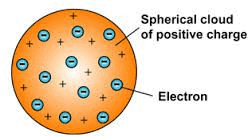Fig: Thomson's atomic model

Limitations of Thomson’s Atomic Model:

1. It could not explain the origin of spectral series of hydrogen and other atoms.

2. It could not explain large angle scattering of α – particles.

Rutherford’s Atomic Model:

On the basis of this experiment, Rutherford made following observations:-

(i) The entire positive charge and almost entire mass of the atom is concentrated at its centre in a very tiny region of the order of 10-15 m, called nucleus.

(ii) The negatively charged electrons revolve around the nucleus in different orbits.

(iii) The total positive charge 011 nucleus is equal to the total negative charge on electron. Therefore atom as a overall is neutral.

(iv) The centripetal force required by electron for revolution is provided by the electrostatic force of attraction between the electrons and the nucleus.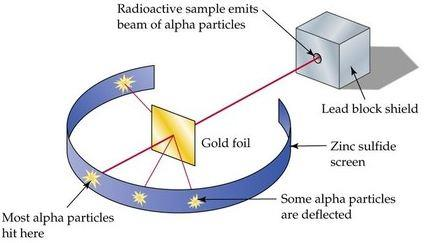Fig: Rutherford's Atomic Model

Distance of Closest Approach:

ro = 1 / 4π εo . 2Ze2 / Ek

where, Ek = kinetic energy of the cc-particle.

Impact Parameter:

The perpendicular distance of the velocity vector of a-particle from the central line of the nucleus, when the particle is far away from the nucleus is called impact parameter.

Impact parameter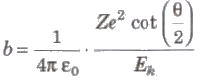where, Z = atomic number of the nucleus, Ek = kinetic energy of the c-particle and θ = angle of scattering.

Rutherford’s Scattering Formula: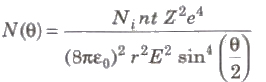where, N(θ) =number of c-particles, Ni = total number of α-particles reach the screen. n = number of atoms per unit volume in the foil, Z = atoms number, E = kinetic energy of the alpha particles and t = foil thickness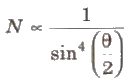Limitations of Rutherford Atomic Model:

(i) About the Stability of Atom: According to Maxwell’s electromagnetic wave theory electron should emit energy in the form of electromagnetic wave during its orbital motion. Therefore. radius of orbit of electron will decrease gradually and ultimately it will fall in the nucleus.

(ii) About the Line Spectrum: Rutherford atomic model cannot explain atomic line spectrum.

Offer running on EduRev: Apply code STAYHOME200 to get INR 200 off on our premium plan EduRev Infinity!

,

,

,

,

,

,

,

,

,

,

,

,

,

,

,

,

,

,

,

,

,

;